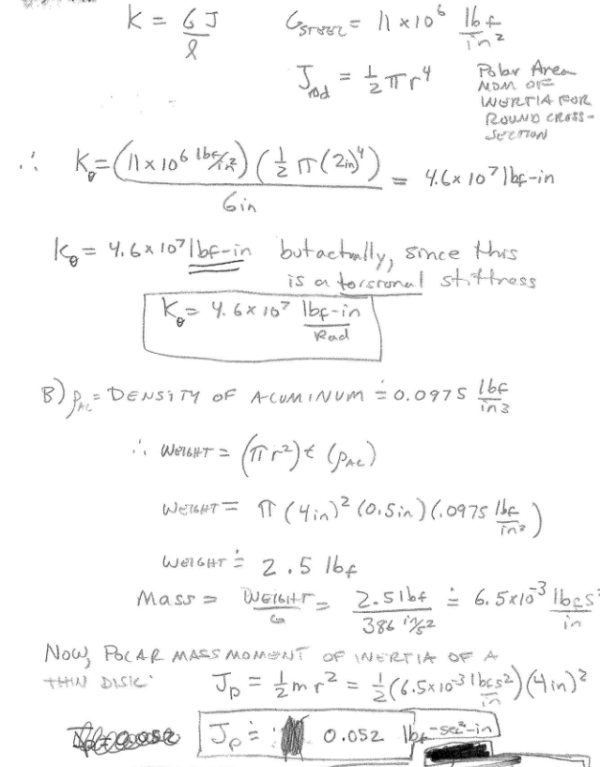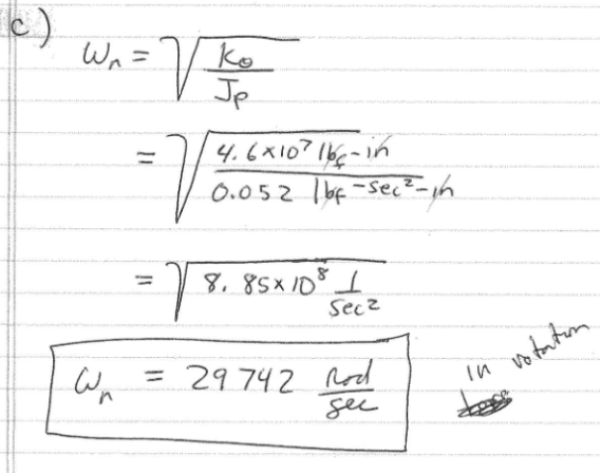It is important to understand the difference between area moment of inertia and mass MOI and when to use each. Do the following: A) From 1 C) above, if the steel rod has a radius=2 in, and L=6 in, what is the torsional stiffness of the rod, Krod? B) from 1 C) above, if the radius of the aluminum wheel is 4 inches, and the wheel thickness is t=0.5 in, what is Jp? C) Using these values, determine the undamped natural frequency of the system.It is important to understand the difference between area moment of inertia and mass MOI and when to use each. Do the following: A) From 1 C) above, if the steel rod has a radius=2 in, and L=6 in, what is the torsional stiffness of the rod, Krod? B) from 1 C) above, if the radius of the aluminum wheel is 4 inches, and the wheel thickness is t=0.5 in, what is Jp? C) Using these values, determine the undamped natural frequency of the system.

Vibrations Page 1 vibrations vibrations vibrations vibrations vibrations vibrations vibrations Vibrations Page 2